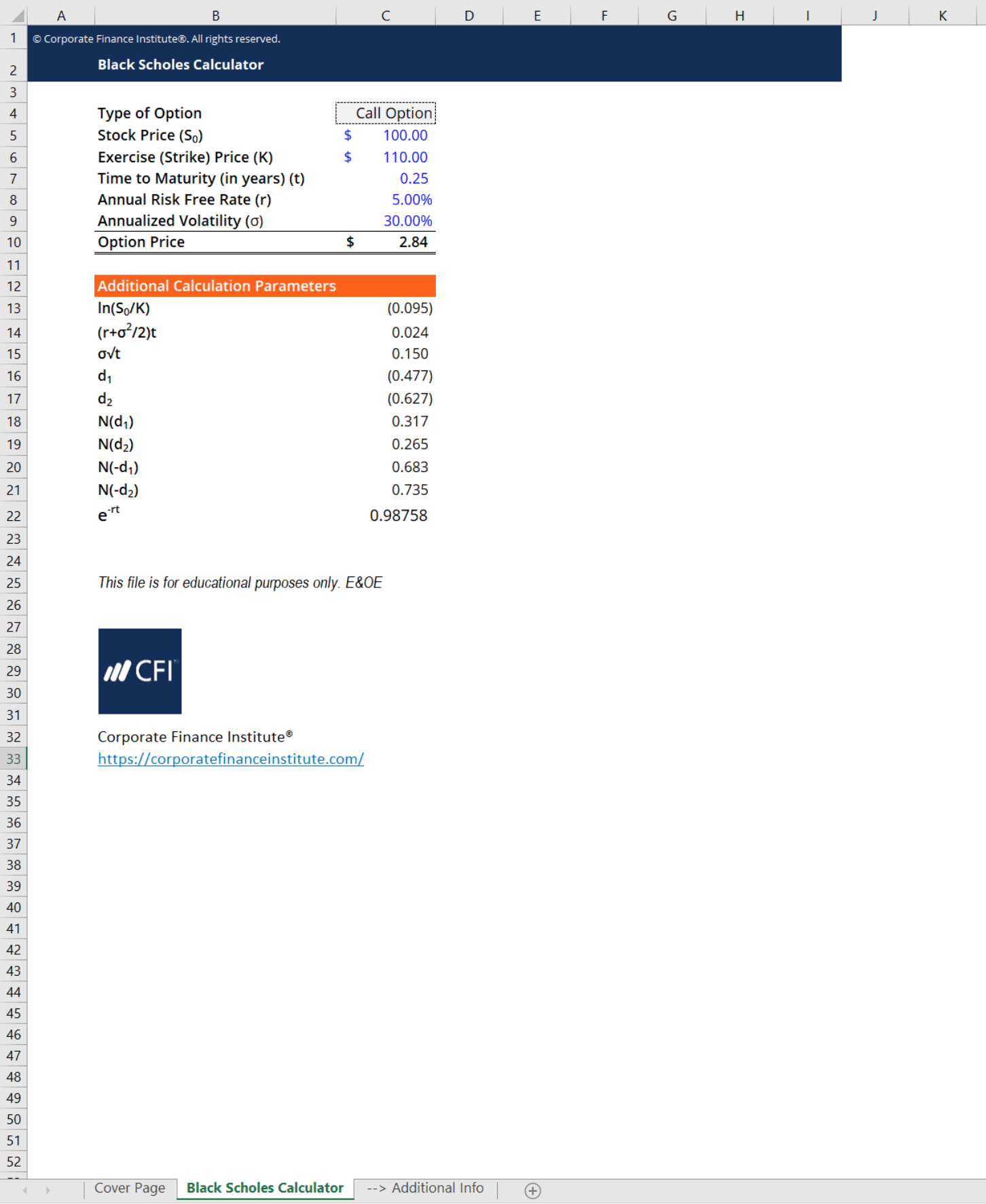# Black Scholes Calculator

## Black Scholes Calculator

This Black Scholes calculator uses the Black-Scholes option pricing method to help you calculate the fair value of a call or put option.

Here is a brief preview of CFI’s Black Scholes calculator.### Option Pricing

CFI’s Black Scholes calculator uses the Black-Scholes option pricing method. Other option pricing methods include the binomial option pricing model and the Monte-Carlo simulation.

The Black-Scholes option pricing method focuses purely on European options on stocks. European options, which can only be exercised on the expiry date of the option. American options, which can be exercised early, cannot be priced using the Black-Scholes option pricing method.

Using this method, the Black Scholes calculator makes a few assumptions that you will need to remember:

• The stock pays no dividends
• Continuously compounded returns are independent over time and are normally distributed
• The volatility of continuously compounded returns is constant and given
• Risk-free rate is constant and given
• Transaction costs or taxes are 0
• Short-selling is possible at no cost
• Borrowing is done at the risk-free rate

The main variables calculated and used in the Black Scholes calculator are:

• Stock Price (S): the price of the underlying asset or stock
• Strike Price (K): the exercise price of the option
• Time to Maturity (t): the time in years until the exercise/maturity date of the option
• Risk-free Rate (r): the risk-free interest rate
• Volatility (σ): the measure of how much the underlying asset’s prices will move over time. This calculator uses annualized volatility

### More Free Templates

For more resources, check out our business templates library to download numerous free Excel modeling, PowerPoint presentation and Word document templates.

• Excel Modeling Templates
• PowerPoint Presentation Templates
• Transaction Document Templates
• CFI Template Marketplace

### Financial Analyst Certification

Become a certified Financial Modeling and Valuation Analyst (FMVA)® by completing CFI’s online financial modeling classes and training program!